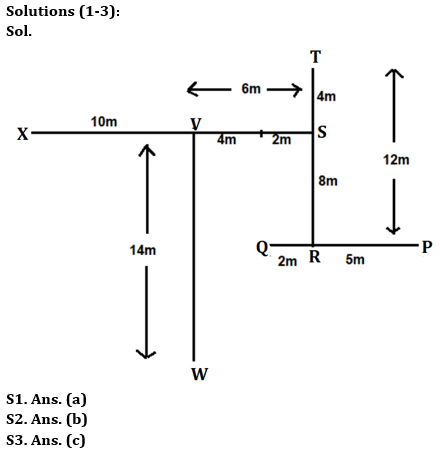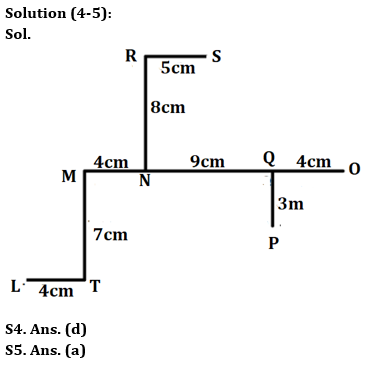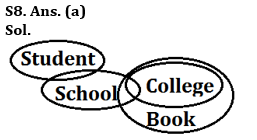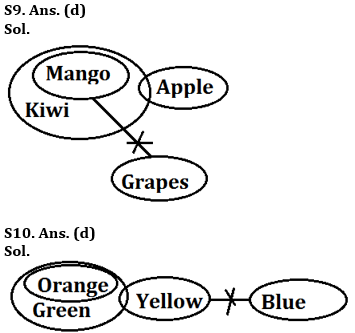Latest Banking jobs   »

# Reasoning Ability Quiz For Bank Foundation 2023 -21st May

Directions (1-3): Answer the questions based on the information given below.
Point Q is 7m to the west of point P. Point R is 12m to the south of point T. Point P is 5m to the east of point R. Point S is 8m to the north of point R. Point V is 6m to the west of point S. Point W is 14m to the south of point V. Point V is 10m east of point X.

Q1. Point X is in which direction and how far with respect to the point S?
(a) 16m, west
(b) 16m, east
(c) 10m, east
(d) 12m, east
(e) 14m, west

Q2. Point W is in which direction with respect to Point T?
(a) South-east
(b) South-west
(c) North-east
(d) North-west
(e) None of the above

Q3. If Kunal sits 4m to the west of point Q, then what will be the shortest distance that Kunal has to cover to reach point S also in which direction?
(a) 10m, north
(b) 12m, south
(c) 10m, north-east
(d) 12m, south-west
(e) None of the above

Directions (4-5): Answer the questions based on the information given below.
Nine points are drawn on a rectangular paper. M is 7cm north of T. L is 4cm west of T. N is 4cm east of M. P is 3cm south of Q. O is 4cm east of Q. S is east of R. N is 8cm south of R. Q is 9cm east of N. The distance between R and S is equal to the shortest distance between O and P.

Q4. Point S is in which direction with respect to Point Q?
(a) South-east
(b) South-west
(c) North-east
(d) North-west
(e) None of the above

Q5. Point P is in which direction with respect to Point M?
(a) South-east
(b) South-west
(c) North-east
(d) North-west
(e) None of the above

Q6. If it is possible to make only one meaningful word with the 3rd, 6th, 8th and 10th letters of the word ‘COMPLIMENT’ (using each letter once), which would be the third letter of the word from the left? If more than one such word can be formed give ‘Y’ as the answer. If no such word can be formed, give ‘Z’ as your answer.
(a) Y
(b) T
(c) I
(d) E
(e) Z

Q7. If in the given number “546839271”, 1 is added to first, third, fifth, seventh and ninth digit and 1 is subtracted from the second, fourth, sixth and eighth digits, then all digits are arranged in descending order from left to right. Which of the following digit is 4th from the right end?
(a) 7
(b) 6
(c) 3
(d) 4
(e) None of these

Directions (8-10): In each of the questions below, some statements are given followed by some Conclusions. You have to take the given statements to be true even, if they seem to be at variance from commonly known facts. Read all the conclusions and then decide which of the given conclusions logically follows from the given statements disregarding commonly known facts.

Q8. Statements: Some Student is School.
Only a few School is College.
All College are Book.
Conclusions: I. All Student is Book is a possibility
II. Some Book is School is a possibility.
III. No Student is College
(a) Only I follows
(b) Only II follows
(c) Only III follows
(d) Both II and III follow
(e) None of the above

Q9. Statements: All Mango are Kiwi.
Only a few Kiwi is Grapes.
No Mango is Apple.
Conclusions: I. No Apple is Grapes
II. All Grapes are Mango is a possibility.
III. All Kiwi are Grapes is a possibility.
(a) Only I follows
(b) Both I and II follow
(c) Both I and III follow
(d) Only II follows
(e) None follows

Q10. Statements: All Orange are Green.
Some Green is Yellow.
No Yellow is Blue.
Conclusions: I. All Orange is Blue.
II. All Green is Blue
III. Some Orange is not Blue.
(a) Only I follows
(b) Only II follows
(c) Only III follows `
(d) Either I or III follows
(e) None of these

SolutionsS6. Ans. (a)
Sol. TIME, EMIT, ITEM

S7. Ans. (d)
Sol. Original Number- 546839271
Obtained Number- 877664332## FAQs

### What is the selection process of the Bank Clerk?

The selection process of the Bank Clerk is Prelims & Mains.

#### Congratulations!Union Budget 2023-24: Free PDF